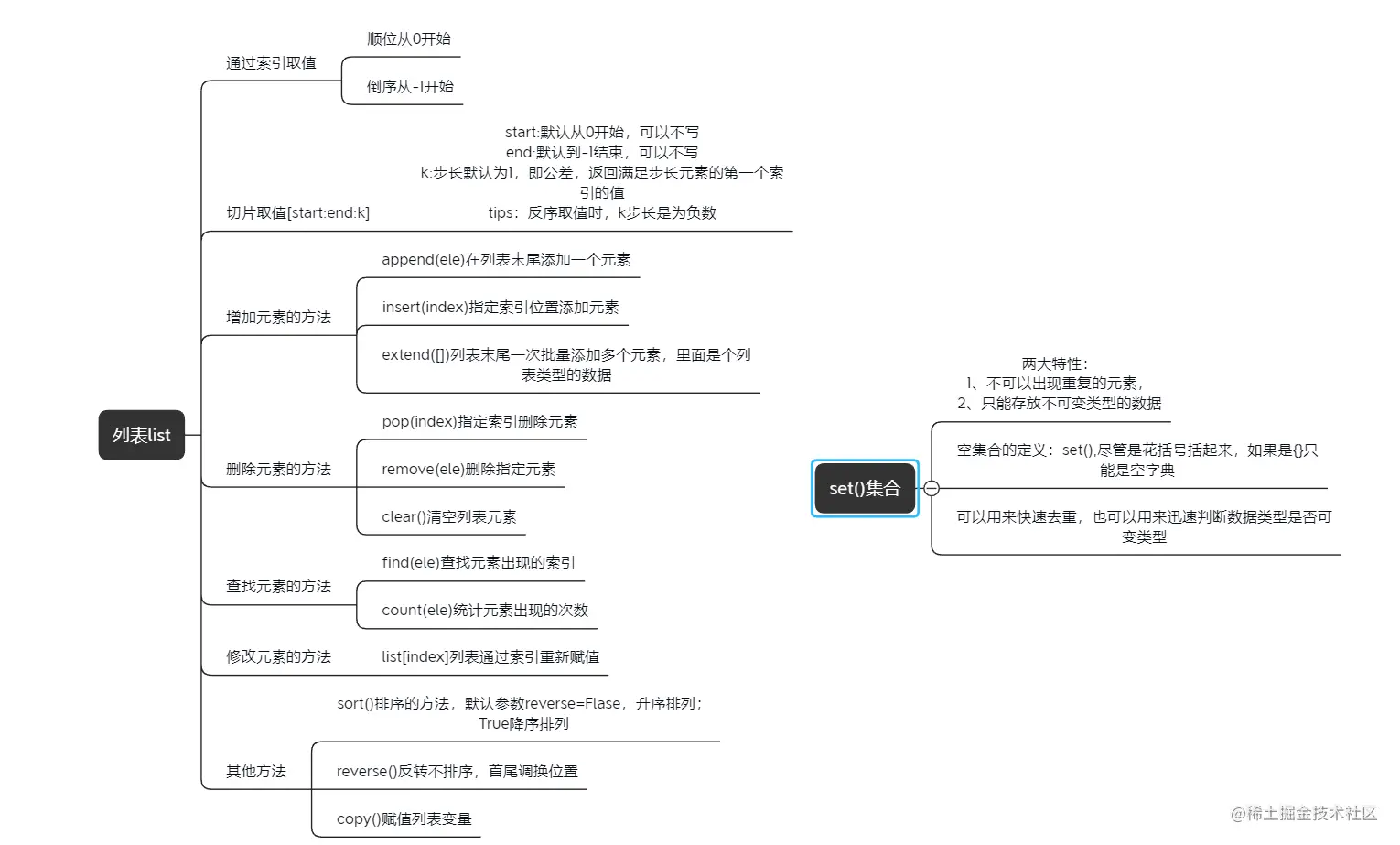# Python interface automation test framework (basic) -- common data types list & set ()

2022-01-31 03:40:36

「 This is my participation 11 The fourth of the yuegengwen challenge 5 God , Check out the activity details ：2021 One last more challenge

## Preface

The next two articles are about variable data types , This paper first list, It is python The most basic data type in , Its elements can be any python data type## list

keyword ：list, Symbol [], Data composition ： Can contain any data type ：int float str list tuple dict Boolean value ; Separate the different elements with commas

#### Create a list of

``````str1="123"

list_1=[]  #  Represents an empty list
list_2=list(str1) #  Split string , A list of elements []

print(list_2) #  Output ：["1","2","3"]
Copy code ``````

#### Value method

• According to the index value
• Support slice value [m:n:k]

• There are many ways to increase ,append Additional , stay list The last addition
``````list1=[1,2,3,4]

list1.append("value")  #  Append... Directly after the list ,
print(list1) #  Output ：[1,2,3,4,"value"]
Copy code ``````
• insert Insert an element into the specified subscript position , The element in its original position moves back
``````list1=[1,2,3,4]

list1.insert(2,"element")

print(list1) #  Output ：[1,2,"element",3,4]
Copy code ``````
• extend(), Parameters are iteratable objects , Such as ：set()、str、tuple、list
``````list1=[1,2,3,4]
list1.extend("abc")

print(list1) #  Output ：[1,2,3,4,"a","b","c"]
Copy code ``````
• Several ways to delete
``````pop()  #  Delete list The last element in the list
remove(element)#  Delete the first occurrence of the element
del list #  Delete list 1 Elements of index location
clear() #  clear list
Copy code ``````
• The assignment operation , That is, replacement :list[index_num]=New_value,index_num The value of the position is replaced with New_value

#### Sort

• Ascending ; Pay attention to the of lifting sequence list Element must be int type
``````list1=[5,2,3]
list1.sort() #  Default ascending order ;

print(list1) #  Output ：[2,3,5]
Copy code ``````
• Descending ,sort, It can be determined by parameters , The default is False Ascending
``````list1=[5,2,3]

list1.sort(reverse=True)

print(list1) #  Output ：[5,3,2]
Copy code ``````
• trans
``````list1=[1,2,3]
list1.reverse()

print(list1) #  Output ：[3,2,1]
Copy code ``````

#### Other built-in functions

• all() # list As long as there is one element in the element list false Then return to false
• any() # list As long as there is one in the element list true Then return to true
• len() # Find the length of list elements
• count() # Calculation list Number of elements
• max() # return list The largest value in the element
• min() # return list The smallest of the elements

## aggregate

set(), Curly braces {} Express , Why talk to list Type one piece , Because it can be in list Switch between , And right list Elements have the property of de duplication

#### establish set() aggregate

``````s=set()  #  Represents an empty set

print(s) #  Output ：set().  Why not {}, because {} It's not worth it dict type

s=set([1,2,3])

print(s) #  Output ：{1,2,3}
Copy code ``````

#### Additive elements , If there are duplicate elements that are overwritten

``````se={1,2,3}

print(se) #  Output ：{1,2,3,4}
Copy code ``````
• It is an immutable data type